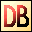DelphiBasics
 LengthFunction Return the number of elements in an array or string System unit
 1 function Length(const SourceString string):Integer; 2 function Length (const  SourceArray array:Integer;
Description
The Length function returns either the number of characters in SourceString, or the number of elements in SourceArray.

It is often used to loop around all characters in a string or elements in an array.
Notes
Arrays start at index = 0 by default. So the length of such an array is 1 more than the highest index.

The length of a Multi-dimensional array is always that of the first subarray - the left most defined dimension.
Related commands
 Copy Create a copy of part of a string or an array SetLength Changes the size of a string, or the size(s) of an array Slice Creates a slice of an array as an Open Array parameterDownload this web site as a Windows program.

 Example code : Get the length of arrays and a string var   openArray  : array of char;   fixedArray : array[2..4] of Integer;   multiArray : array[2..4, 1..9] of Integer;   shortStr   : shortstring;   longStr    : string;   i          : Integer; begin   // Define the length of the open array   SetLength(openArray, 17);   // Show the length of the arrays   ShowMessage('Length of openArray = '+IntToStr(Length(openArray)));   ShowMessage('Length of fixedArray = '+IntToStr(Length(fixedArray)));   ShowMessage('Length of multiArray = '+IntToStr(Length(multiArray)));   // Assign to the strings   shortStr := 'ABCDEFGH';   longStr  := '12345678901234567890';   ShowMessage('Length of shortStr = '+IntToStr(Length(shortStr)));   ShowMessage('Length of longStr = '+IntToStr(Length(longStr)));   // Display one letter at a time from the short string   for i := 1 to Length(shortStr) do     ShowMessage('Letter '+IntToStr(i)+' = '+shortStr[i]); end; Show full unit code Length of openArray = 17   Length of fixedArray = 3   Length of multiArray = 3   Length of shortStr = 8   Length of longStr = 20   Letter 1 = A   Letter 2 = B   Letter 3 = C   Letter 4 = D   Letter 5 = E   Letter 6 = F   Letter 7 = G   Letter 8 = H